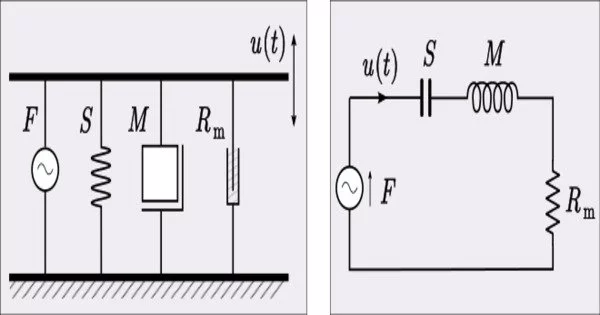Engineering

# Impedance AnalogyThe impedance analogy is a method of representing a mechanical system with an electrical system that is analogous. The concept of using an electrical analogy to understand and analyze systems with impedance in non-electrical domains is referred to as impedance analogy. The benefit of doing so is that there is a large body of theory and analysis techniques concerning complex electrical systems, particularly filters.

Impedance is a measure of resistance to the flow of a specific quantity in a system, such as electric current or sound waves. It is made up of both resistance and reactance. These tools in the electrical domain can be directly applied to a mechanical system without modification by converting to an electrical representation. In electromechanical systems, converting the mechanical part of the system into the electrical domain allows the entire system to be analyzed as a unified whole.

Using analogous electrical circuits and the impedance analogy, you can represent systems in various fields such as fluid dynamics, acoustics, and heat transfer. This enables you to apply well-established electrical engineering principles and techniques to analyze and solve problems in these various domains.

The mathematical behavior of the simulated electrical system is the same as that of the represented mechanical system. Each element in the electrical domain has a mechanical domain corresponding element with an analogous constitutive equation. All circuit analysis laws that apply in the electrical domain, such as Kirchhoff’s circuit laws, also apply in the mechanical impedance analogy.

Let’s consider a few examples:

• Fluid Dynamics: An electrical circuit analogy can be used to represent fluid flow in fluid systems such as pipes or channels. The flow rate represents the electric current, the pressure represents the voltage, and the resistance of the pipe represents the viscosity of the fluid. Analogous circuit elements such as resistors, capacitors, and inductors can be used to analyze and predict the behavior of the fluid system.
• Acoustics: Sound waves can be represented in acoustics by an analogous electrical circuit. The sound pressure is equivalent to voltage, the sound source is equivalent to a current source, and the acoustic impedance represents the resistance to the flow of sound waves. The transmission and reflection of sound waves through various media and structures can be studied using impedance analogy.
• Heat Transfer: The impedance analogy can be used to represent heat conduction in solid objects. Temperature represents voltage in this case, heat flux represents current, and thermal resistance represents the resistance to heat flow. Heat transfer in complex systems can be analyzed and temperature distributions predicted using analogous electrical circuit elements.

The impedance analogy provides a powerful framework for understanding and analyzing systems in different domains. It allows engineers and scientists to leverage their knowledge of electrical circuits and apply it to other areas, facilitating the analysis, design, and optimization of various systems.